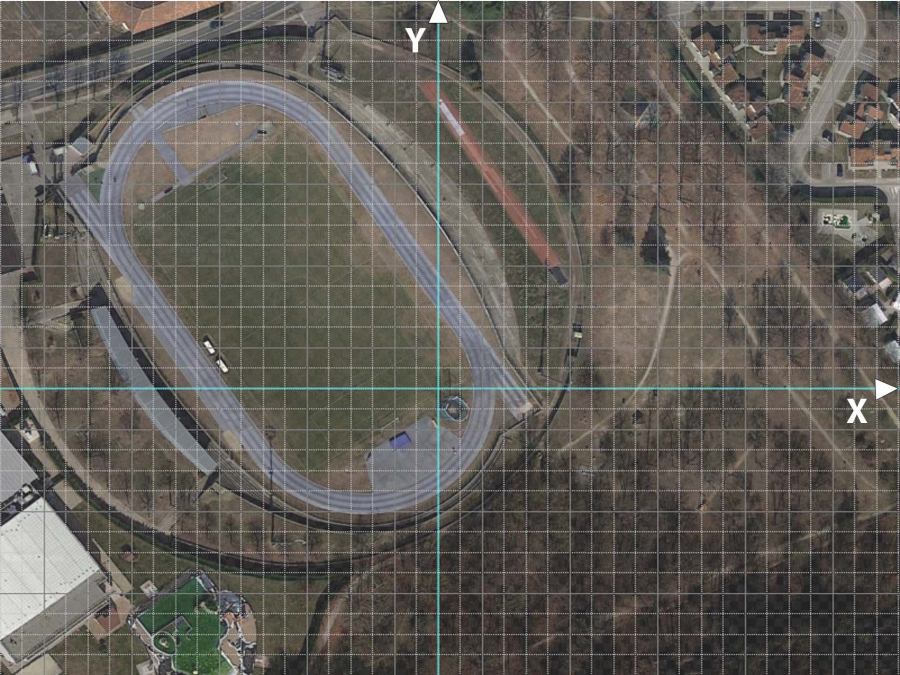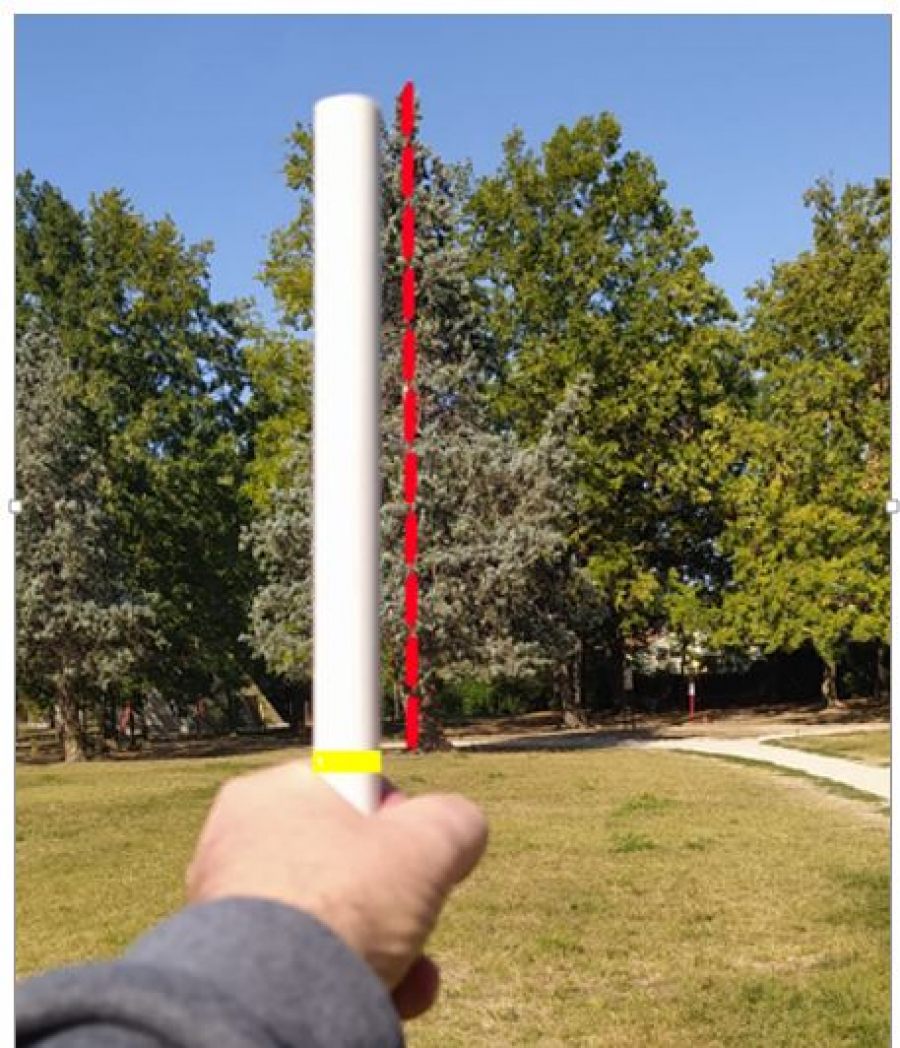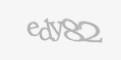Sunday, 29 January 2023 09:36

## TREE HUNTING

Rate this item
(1 Vote)
|||| ||||

Could you measure and identify a tree in a park?

Starting from a map analysis, couples of students will calculate the tree measurement point in a park. Then, they will have to join the measurement point and discover how much the tree is high applying simple math rules. Finally they will have to recognise the tree specie using mobile apps and compare results with their classroom mates.

• What you will learn:
• Science
• - Observe and recognise different species of trees
•
• Technology
• - Use mobile apps to geolocalizate a point
• - Use mobile apps to recognize trees
•
• Math:
• - How to work with equations of the line
• - How to use math to solve simple real life problems.
• Expected Time:

Less than 5 hours

• Materials & Recycled Materials:
• - Map
• - Cartesian plane
• - Tape measure
• - Sheets of paper and pen
• - Wooden or plastic stick
• Guide:
• 1) Print the map of the working area, overlapped with a cartesian plane
•
••
• 2) Solve problem number 1: write the equation of the line passing through two points, for example A(5;2) B(14;6). Each couple of studens will have different points data.
•
••
• 3) Determine the measurement point C(x;y) by solving the following linear system (system of two equations in the two variables)
•
• Equation 1 is the equation of the straight line of point 1
• Equation 2 is:  y=1/2 x+1
•
• 4) Draw on the Cartesian plane (file cartesian-plane.jpg) the point C (x;y) solution of the linear system of point 2). Try to identify the point coordinates on Google Maps
• 5) Go to the place identified on the map (point C(x;y)). In that place you have to find the assigned tree.
• 6) Using the stick (plastic or wooden) and the measuring wheel measure the tree height using the arc of a circle technique .
•
••
••
• 7) Using your experience and with the help of a moblie app like Plantnet, try to identify the tree specie.
• 8) Once returned in the school, compare the results with the other groups. Do you have identified the same tree specie? Do you have the same result of the tree height? Take the mathematical average of all the results, in order to reduce measurement errors!
• Further Steps:

If you want more fun you can:

• - use systems of equations with line and parable
• - use GPS Coordinates - Latitude and Longitude - instead of Cartesian plane
• Software used in the project:
• - Plantnet App
• Difficulty level: Easy
• Developed By: Apro Formazione - IT

### Media

No media available for this project